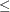### Example 6.6 Migration to OPTMODEL: Generalized Networks

The following example shows how to use PROC OPTMODEL to solve the example Generalized Networks: Using the EXCESS= Option in Chapter 6: The NETFLOW Procedure in SAS/OR 12.3 User's Guide: Mathematical Programming Legacy Procedures. The input data sets are the same as in the PROC NETFLOW example.

```title 'Generalized Networks';

data garcs;
input _from_ \$ _to_ \$ _cost_ _mult_;
datalines;
s1 d1 1 .
s1 d2 8 .
s2 d1 4 2
s2 d2 2 2
s2 d3 1 2
s3 d2 5 0.5
s3 d3 4 0.5
;
```
```data gnodes;
input _node_ \$ _sd_ ;
datalines;
s1 5
s2 20
s3 10
d1 -5
d2 -10
d3 -20
;
```

The following PROC OPTMODEL statements read the data sets, build the linear programming model, solve the model, and output the optimal solution to a SAS data set called `GENETOUT`:

```proc optmodel;
set <str> NODES;
num _sd_ {NODES} init 0;
read data gnodes into NODES=[_node_] _sd_;

set <str,str> ARCS;
num _lo_ {ARCS} init 0;
num _capac_ {ARCS} init .;
num _cost_ {ARCS};
num _mult_ {ARCS} init 1;
read data garcs nomiss into ARCS=[_from_ _to_] _cost_ _mult_;
NODES = NODES union (union {<i,j> in ARCS} {i,j});
```
```   var Flow {<i,j> in ARCS} >= _lo_[i,j];
min obj = sum {<i,j> in ARCS} _cost_[i,j] * Flow[i,j];
con balance {i in NODES}: sum {<(i),j> in ARCS} Flow[i,j]
- sum {<j,(i)> in ARCS} _mult_[j,i] * Flow[j,i] = _sd_[i];

num infinity = min {r in {}} r;
/* change equality constraint to le constraint for supply nodes */
for {i in NODES: _sd_[i] > 0} balance[i].lb = -infinity;

solve;

num _supply_ {<i,j> in ARCS} = (if _sd_[i] ne 0 then _sd_[i] else .);
num _demand_ {<i,j> in ARCS} = (if _sd_[j] ne 0 then -_sd_[j] else .);
num _fcost_ {<i,j> in ARCS} = _cost_[i,j] * Flow[i,j].sol;

create data gnetout from [_from_ _to_]
_cost_ _capac_ _lo_ _mult_ _supply_ _demand_ _flow_=Flow _fcost_;
quit;
```

To solve a generalized network flow problem, the usual balance constraint is altered to include the arc multiplier _mult_[i,j] in the second sum. The balance constraint is initially declared as an equality, but to mimic the EXCESS=SUPPLY option in PROC NETFLOW, the sense of this constraint is changed toby relaxing the constraint’s lower bound for supply nodes. The output data set is displayed in Output 6.6.1.

Output 6.6.1: Optimal Solution with Excess Supply

Obs _from_ _to_ _cost_ _capac_ _lo_ _mult_ _supply_ _demand_ _flow_ _fcost_
1 s1 d1 1 . 0 1.0 5 5 5 5
2 s1 d2 8 . 0 1.0 5 10 0 0
3 s2 d1 4 . 0 2.0 20 5 0 0
4 s2 d2 2 . 0 2.0 20 10 5 10
5 s2 d3 1 . 0 2.0 20 20 10 10
6 s3 d2 5 . 0 0.5 10 10 0 0
7 s3 d3 4 . 0 0.5 10 20 0 0

The log is displayed in Output 6.6.2.

Output 6.6.2: OPTMODEL Log

 NOTE: There were 6 observations read from the data set WORK.GNODES. NOTE: There were 7 observations read from the data set WORK.GARCS. NOTE: Problem generation will use 4 threads. NOTE: The problem has 7 variables (0 free, 0 fixed). NOTE: The problem has 6 linear constraints (3 LE, 3 EQ, 0 GE, 0 range). NOTE: The problem has 14 linear constraint coefficients. NOTE: The problem has 0 nonlinear constraints (0 LE, 0 EQ, 0 GE, 0 range). NOTE: The OPTMODEL presolver is disabled for linear problems. NOTE: The LP presolver value AUTOMATIC is applied. NOTE: The LP presolver removed 2 variables and 2 constraints. NOTE: The LP presolver removed 4 constraint coefficients. NOTE: The presolved problem has 5 variables, 4 constraints, and 10 constraint coefficients. NOTE: The LP solver is called. NOTE: The Dual Simplex algorithm is used. Objective Phase Iteration        Value         Time D 1          1    0.000000E+00         0 D 2          2    1.500000E+01         0 D 2          4    2.500000E+01         0 NOTE: Optimal. NOTE: Objective = 25. NOTE: The Dual Simplex solve time is 0.05 seconds. NOTE: The data set WORK.GNETOUT has 7 observations and 10 variables.

Now consider the previous example but with a slight modification to the arc multipliers, as in the PROC NETFLOW example:

```data garcs1;
input _from_ \$ _to_ \$ _cost_ _mult_;
datalines;
s1 d1 1 0.5
s1 d2 8 0.5
s2 d1 4 .
s2 d2 2 .
s2 d3 1 .
s3 d2 5 0.5
s3 d3 4 0.5
;
```

The following PROC OPTMODEL statements are identical to the preceding example, except for the balance constraint. The balance constraint is still initially declared as an equality, but to mimic the PROC NETFLOW EXCESS=DEMAND option, the sense of this constraint is changed toby relaxing the constraint’s upper bound for demand nodes.

```proc optmodel;
set <str> NODES;
num _sd_ {NODES} init 0;
read data gnodes into NODES=[_node_] _sd_;

set <str,str> ARCS;
num _lo_ {ARCS} init 0;
num _capac_ {ARCS} init .;
num _cost_ {ARCS};
num _mult_ {ARCS} init 1;
read data garcs1 nomiss into ARCS=[_from_ _to_] _cost_ _mult_;
NODES = NODES union (union {<i,j> in ARCS} {i,j});

var Flow {<i,j> in ARCS} >= _lo_[i,j];
for {<i,j> in ARCS: _capac_[i,j] ne .} Flow[i,j].ub = _capac_[i,j];
min obj = sum {<i,j> in ARCS} _cost_[i,j] * Flow[i,j];
con balance {i in NODES}: sum {<(i),j> in ARCS} Flow[i,j]
- sum {<j,(i)> in ARCS} _mult_[j,i] * Flow[j,i] = _sd_[i];

num infinity = min {r in {}} r;
/* change equality constraint to ge constraint */
for {i in NODES: _sd_[i] < 0} balance[i].ub = infinity;

solve;

num _supply_ {<i,j> in ARCS} = (if _sd_[i] ne 0 then _sd_[i] else .);
num _demand_ {<i,j> in ARCS} = (if _sd_[j] ne 0 then -_sd_[j] else .);
num _fcost_ {<i,j> in ARCS} = _cost_[i,j] * Flow[i,j].sol;

create data gnetout1 from [_from_ _to_]
_cost_ _capac_ _lo_ _mult_ _supply_ _demand_ _flow_=Flow _fcost_;
quit;
```

The output data set is displayed in Output 6.6.3.

Output 6.6.3: Optimal Solution with Excess Demand

Obs _from_ _to_ _cost_ _capac_ _lo_ _mult_ _supply_ _demand_ _flow_ _fcost_
1 s1 d1 1 . 0 0.5 5 5 5 5
2 s1 d2 8 . 0 0.5 5 10 0 0
3 s2 d1 4 . 0 1.0 20 5 0 0
4 s2 d2 2 . 0 1.0 20 10 5 10
5 s2 d3 1 . 0 1.0 20 20 15 15
6 s3 d2 5 . 0 0.5 10 10 0 0
7 s3 d3 4 . 0 0.5 10 20 10 40

The log is displayed in Output 6.6.4.

Output 6.6.4: OPTMODEL Log

 NOTE: There were 6 observations read from the data set WORK.GNODES. NOTE: There were 7 observations read from the data set WORK.GARCS1. NOTE: Problem generation will use 4 threads. NOTE: The problem has 7 variables (0 free, 0 fixed). NOTE: The problem has 6 linear constraints (0 LE, 3 EQ, 3 GE, 0 range). NOTE: The problem has 14 linear constraint coefficients. NOTE: The problem has 0 nonlinear constraints (0 LE, 0 EQ, 0 GE, 0 range). NOTE: The OPTMODEL presolver is disabled for linear problems. NOTE: The LP presolver value AUTOMATIC is applied. NOTE: The LP presolver removed 2 variables and 2 constraints. NOTE: The LP presolver removed 4 constraint coefficients. NOTE: The presolved problem has 5 variables, 4 constraints, and 10 constraint coefficients. NOTE: The LP solver is called. NOTE: The Dual Simplex algorithm is used. Objective Phase Iteration        Value         Time D 1          1    0.000000E+00         0 D 2          2    4.997000E+01         0 D 2          5    7.000000E+01         0 NOTE: Optimal. NOTE: Objective = 70. NOTE: The Dual Simplex solve time is 0.05 seconds. NOTE: The data set WORK.GNETOUT1 has 7 observations and 10 variables.